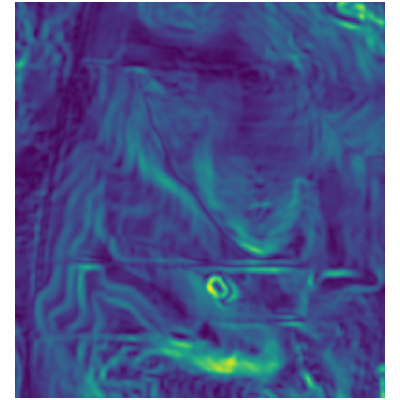## NAME

r.tri - Computes the Terrain Ruggedness Index.

## KEYWORDS

raster, surface, terrain, ruggedness, parallel

## SYNOPSIS

r.tri
r.tri --help
r.tri [-c] input=name output=name size=integer [exponent=float] [processes=integer] [--overwrite] [--help] [--verbose] [--quiet] [--ui]

### Flags:

-c
Use circular neighborhood
--overwrite
Allow output files to overwrite existing files
--help
Print usage summary
--verbose
Verbose module output
--quiet
Quiet module output
--ui
Force launching GUI dialog

### Parameters:

input=name [required]
Input elevation raster
output=name [required]
Output Terrain Ruggedness Index (TRI)
size=integer [required]
Size of neighbourhood in cells (> 2 and <= 51)
Default: 3
exponent=float
Distance weighting exponent (>= 0 and <= 4.0)
Default: 0.0
processes=integer
Number of processing cores for tiled calculation
Number of processing cores for tiled calculation (negative numbers are all cpus -1, -2 etc.)
Default: 1

## DESCRIPTION

r.tri calculates the Terrain Ruggedness Index (TRI) of Riley et al. (1999). The index represents the mean change in elevation between a grid cell and its neighbours, over a user-specified moving window size. The original calculation in Riley et al. (1999) used only a 3x3 neighbourhood and represented the sum of the absolute deviations between the center pixel and its immediate 8 neighbours. In r.tri, this calculation is modified so that the calculation can be extended over any scale by taking the mean of the absolute deviations.

## NOTES

r.tri produces fairly similar results to the average deviation of elevation values, apart from the center pixel is used in place of the mean. In practice, this produces a slightly less smoothed result that can potentially highlight finer-scale terrain features. However, because the terrain ruggedness index does not consider the distance of each cell from the centre cell in it's calculation, the TRI results can become noisy with large window sizes. To avoid this, weighting each cell by the inverse of its distance can be used by setting the exponent parameter to > 0.

Similar to many other GRASS GIS algorithms, cell padding is not performed automatically which will leave null values at the boundaries of the output raster relative to the size of the input raster. To minimize this effect the DEM needs to be buffered/grown prior to using r.tri.

Currently, r.tri is implemented using a r.mapcalc focal function. This becomes slow for large window sizes. To reduce computational times for large raster datasets, setting processes parameter to > 1 will use a parallelized and tiled calculations that is spread across multiple processing cores. This option requires the GRASS addon r.mapcalc.tiled to be installed.

## EXAMPLE

```d.rast map=elev_lid792_1m@PERMANENT
g.region raster=elev_lid792_1m@PERMANENT -a
r.tri input=elev_lid792_1m@PERMANENT size=9 output=tri
```## REFERENCES

Riley, S. J., S. D. DeGloria and R. Elliot (1999). A terrain ruggedness index that quantifies topographic heterogeneity, Intermountain Journal of Sciences, vol. 5, No. 1-4, 1999.

r.mapcalc, r.mapcalc.tiled

Steven Pawley

## SOURCE CODE

Available at: r.tri source code (history)

Latest change: Friday Sep 29 17:17:18 2023 in commit: 7a3dfb74e3827a3960a761060449f2ddaf3503e8

© 2003-2023 GRASS Development Team, GRASS GIS 8.3.1dev Reference Manual